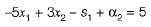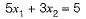Courses

# Test: Linear Programming - 2

## 7 Questions MCQ Test Topicwise Question Bank for Mechanical Engineering | Test: Linear Programming - 2

Description
This mock test of Test: Linear Programming - 2 for Mechanical Engineering helps you for every Mechanical Engineering entrance exam. This contains 7 Multiple Choice Questions for Mechanical Engineering Test: Linear Programming - 2 (mcq) to study with solutions a complete question bank. The solved questions answers in this Test: Linear Programming - 2 quiz give you a good mix of easy questions and tough questions. Mechanical Engineering students definitely take this Test: Linear Programming - 2 exercise for a better result in the exam. You can find other Test: Linear Programming - 2 extra questions, long questions & short questions for Mechanical Engineering on EduRev as well by searching above.
QUESTION: 1

Solution:
QUESTION: 2

Solution:
QUESTION: 3

### A tie for leaving variables in simplex procedure implies

Solution:
QUESTION: 4

The steps followed for the development of linear programming model are
1. state of problem in the form of a linear programming model
2. determine the decision variables
3. write the objective function
4. develop inequations (or equations) for the constraints.
The correct order is

Solution:
QUESTION: 5

A constraint in equation 5x1 - 3x2 ≤ -5 is converted as, (3x2 + α1) - (5x1 + -s1) = 5
Then 's1' is called as

Solution:This is used to convert constraint in equation.Thus s1 is the surplus variable.

QUESTION: 6

Consider the following Linear Programming Problem (LPP):​Solution:
QUESTION: 7

The linear programming is used for optimization problems which satisfy the following conditions:
1. Objective function expressed as a linear function of variables.
2. Resources are unlimited.
3. The decision variables are inter-related and non-negative.
Which of these statements is/are correct?

Solution: